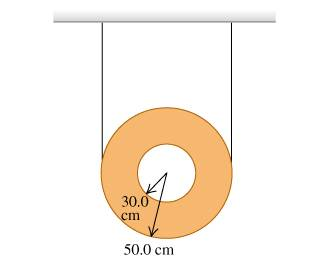# Rotation problem solved with conservation of energy.

• btbam91

#### btbam91

I have a quick problem.

A uniform hollow disk has two pieces of thin light wire wrapped around its outer rim and is supported from the ceiling (the figure ). Suddenly one of the wires breaks, and the remaining wire does not slip as the disk rolls down.Use energy conservation to find the speed of the center of this disk after it has fallen a distance of 1.20 .

So I get.

mgh = .5mv^2 + .5m(r1^2+r2^2)(v^2/x^2)

Okay, so I ran through this problem yesterday and my my problem was determining the value of x in the above equation. Thing is, I found it to be .7 yesterday and popped out the correct answer for the velocity as 3.74 m/s.

The problem today is, I have no idea how I found that x to be .7. It makes no sense because I thought that x represented the distance from the center of the disc to the axis of rotation, which just be .5 no?

This is bugging me dearly, so thanks for the help!

4.5 hours later........

the equation for rotational kinetic energy has a .5 and so does the formula for the moment of inertia. I was missing one of the .5's.

FINALLY!